Expert Modelling: Hands-on help from Rocscience Engineers. Register Here

# Strength Properties

In the Define Material Properties dialog, the Strength Parameters allow you to define:

• The failure (strength) criterion for a material
• The material type (elastic or plastic)

## Failure Criterion

The following strength criteria are available in RS2 for defining the strength of your rock mass or soil:

See below for information about each failure criterion.

Note: for the Mohr-Coulomb, Hoek-Brown or Generalized Hoek-Brown criteria, you can link directly to RSData to help determine values of input parameters.

## Material Type

You may select either Elastic or Plastic for the Material Type.

Elastic Material

If you choose Material Type = Elastic, then the failure criterion parameters which you enter will only be used for the calculation and plotting of strength factor within the material. Although an Elastic material cannot "fail", the failure envelope allows a degree of overstress to be calculated.

Plastic Material

If you choose Material Type = Plastic , the strength parameters you enter will be used in the analysis if yielding occurs. This is unlike Elastic materials, where the strength parameters are only used to obtain values of strength factor, but do not affect the analysis results (i.e. stresses and displacements are not affected).

If you define a material to be Plastic then you may also define residual strength parameters and a dilation parameter, depending on the strength criterion.

• If the residual strength parameters are equal to the peak parameters, then you are defining an "ideally" elastic-plastic material.
• The dilation is a measure of the increase in volume of the material when sheared (see below for more information).

Note: if you define a material as Plastic, then you are restricted to Isotropic elastic properties for that material. You cannot combine plasticity with Transversely Isotropic or Orthotropic elastic properties.

## Mohr-Coulomb

For the Mohr-Coulomb criterion you must define the following parameters:

• Cohesion
• Friction Angle
• Tensile Strength

If you are not considering pore pressure in the analysis, then the cohesion and friction angle are total stress parameters. If you are considering pore pressure, then cohesion and friction angle are effective stress parameters.

If the Material Type = Plastic, you will also be able to define:

• Dilation Angle
• Residual values of cohesion, friction angle and tensile strength

For assistance with determining Mohr-Coulomb parameters you can startup RSData by selecting thebutton, and paste applicable data from RSData by selecting the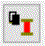button. See below for further information.

## Hoek-Brown

The Hoek-Brown strength criterion in RS2, refers to the ORIGINAL Hoek-Brown failure criterion [ Hoek & Bray (1981) ], described by the following equation: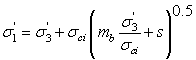Note that this is a special case of the Generalized Hoek-Brown criterion, with the constant a = 0.5. See below for definition of the parameters in this equation.

The original Hoek-Brown criterion has been found to work well for most rocks of good to reasonable quality in which the rock mass strength is controlled by tightly interlocking angular rock pieces.

For lesser quality rock masses, the Generalized Hoek-Brown criterion can be used.

For assistance with determining Hoek-Brown parameters you can startup RSData by selecting thebutton, and paste applicable data from RSData by selecting thebutton. See below for further information.

## Generalized Hoek-Brown

For the Generalized Hoek-Brown criterion you must define the following parameters:

• The intact uniaxial compressive strength (UCS) of the rock.
• parameters mb, s and a

If the Material Type = Plastic, you will also be able to define:

• Dilation parameter
• Residual values of mb, s and a

The Generalized Hoek-Brown strength criterion is described by the following equation: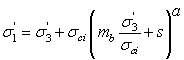where:

• mb is a reduced value (for the rock mass) of the material constant mi (for the intact rock)
• s and a are constants which depend upon the characteristics of the rock mass
•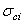is the uniaxial compressive strength (UCS) of the intact rock pieces
•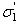and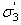are the axial and confining effective principal stresses respectively

In most cases it is practically impossible to carry out triaxial or shear tests on rock masses at a scale which is necessary to obtain direct values of the parameters in the Generalized Hoek-Brown equation. Therefore some practical means of estimating the material constants mb, s and a is required. According to the latest research, the parameters of the Generalized Hoek-Brown criterion [ Hoek, Carranza-Torres & Corkum (2002) ], can be determined from the following equations: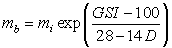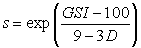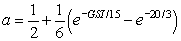where:

• GSI is the Geological Strength Index
• mi is a material constant for the intact rock
• The parameter D is a "disturbance factor" which depends upon the degree of disturbance to which the rock mass has been subjected by blast damage and stress relaxation. It varies from 0 for undisturbed in situ rock masses to 1 for very disturbed rock masses.

Parameter Calculator

The parameters GSI, mi, D and UCS can be estimated for your material using the Parameter Calculator dialog, which is available by selecting the GSI buttonin the Define Material Properties dialog. Values of mb, s and a are automatically calculated from the above equations, and the rock mass modulus is also calculated. See the Parameter Calculator topic for more information.

For assistance with determining Generalized Hoek-Brown parameters, you can startup RSData by selecting thebutton, and paste applicable data from RSData by selecting thebutton. See below for further information.

## Drucker-Prager

The Drucker-Prager strength parameters are:

• Tensile Strength
• q parameter
• k parameter

If the Material Type = Plastic, you will also be able to define:

• Dilation parameter
• q (residual), k(residual)

Note: to calculate equivalent Drucker-Prager parameters based on Mohr-Coulomb parameters, you can use the following equations: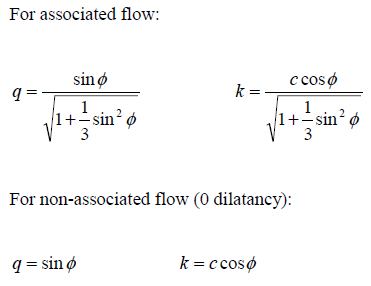## Cam-Clay

Specification of the Cam-Clay model requires five material parameters, and the initial state of consolidation. These parameters are summarized below. For a theoretical overview of the Cam-Clay and Modified Cam-Clay strength models, see the Theory section.

Lambda

Kappa

Critical State Line Slope (M)

The slope (M) of the Critical State Line (CSL) in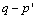space.

Specific Volume (N or Gamma)

There are two possible methods for defining the specific volume parameter. The N parameter defines the specific volume of the normal compression line at unit pressure. The Gamma (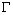) parameter defines the specific volume of the CSL at unit pressure. The choice of parameter can be selected by the user in the Cam-Clay Options dialog (see below).

Elastic Parameters

There are two possible methods of defining the elastic parameter for a Cam-Clay material. You may enter either the Shear Modulus or Poisson’s Ratio. The choice of parameter can be selected by the user in the Cam-Clay Options dialog (see below).

Initial State of Consolidation

There are two possible methods for defining the initial state of consolidation. The Overconsolidation Ratio (OCR) is the ratio of the previous maximum mean stress to the current mean stress. Or you can specify the Preconsolidation Pressure (Po). The choice of parameter can be selected by the user in the Cam-Clay Options dialog (see below).

Cam-Clay Options

If you select the Options button in the Define Material Properties dialog, you will be able to choose the method of defining the following Cam-Clay parameters:

• Elastic Parameters (Shear Modulus or Poisson's Ratio)
• Specific Volume at Unit Pressure (N or Gamma)
• Initial State of Consolidation (OCR or Preconsolidation pressure)

The selections in the Cam-Clay Options dialog determine the Cam-Clay parameters which can be entered in the Define Material Properties dialog, for a given material.

## Modified Cam-Clay

The Modified Cam-Clay strength model in RS2 has the same input parameters as the Cam-Clay model, but uses the Modified Cam-Clay equations. See above for a summary of input parameters.

For a theoretical overview of the Cam-Clay and Modified Cam-Clay strength models, see the Theory section.

## Discrete Function

The Discrete Function option allows you to define Mohr-Coulomb shear strength parameters (cohesion, friction angle) at a grid of discrete points within a material. The shear strength at any point within the material is then interpolated from the data at the grid points. This allows you to define any type of strength distribution within a material. Elastic modulus can also be defined at the discrete grid points.

See the Discrete Strength Function topic for details.

## Mohr-Coulomb with Cap

See the Mohr-Coulomb with Cap document in the theory section for details.

## Softening Hardening Model

See the Softening-Hardening Material Model document in the theory section for details.

## Finn Model

It should be noted that the Finn Model has all of the same parameters as Mohr-Coulomb, but also contains additional Dynamic parameters.

Since the primary effect of liquefaction is the irrecoverable volume contraction in the soil grains, meaning a change in volumetric strain, when the soil is under a strain cycle with constant confining stress. If the voids are filled with fluid, the pore pressure and effective pressure stay constant if the volume is constant; however, when there is a volume contraction, the pore pressure increases and the effective pressure decreases. This independency of the volumetric strain and cyclic shear-strain amplitude with respect to confining stress is noted by Martin et al. (1975) defined this mechanism as the following empirical equation: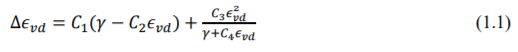Where ∆ϵ vd is the cyclic shear-strain amplitude,

γ is the engineering shear strain,

C 1, C 2 , C 3, and C 4 are constants equal to 0.8, 0.79, 0.45 and 0.73 respectively.

It should be noted that the equation takes account of the accumulated irrecoverable volume strain ϵ vd by decreasing the increment in volume strain as the volume strain is accumulated. Presumably, ∆ϵ vd should be zero if γ is zero. Martipute the change in pore pressure by assuming certain boundary conditions that were not clearly stated, which are taken care by RS2.

Another similar formula derived by Byrne (1991) also define this mechanism, in a simpler way: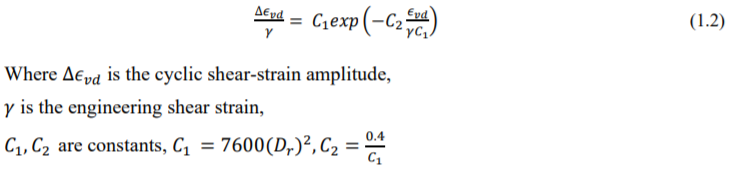## Dilation Parameter

A dilation parameter can be defined for Mohr-Coulomb, Hoek-Brown and Drucker-Prager materials, if the Material Type = Plastic.

Dilatancy is a measure of how much volume increase occurs when the material is sheared.

• For a Mohr-Coulomb material, dilatancy is an angle that generally varies between zero (non-associative flow rule) and the friction angle (associative flow rule).
• For Hoek-Brown materials, dilatancy is defined using a dimensionless parameter that generally varies between zero and m.

Low dilation angles/parameters (i.e. zero) are generally associated with soft rocks while high dilation angles/parameters (i.e. phi or m) are associated with hard brittle rock masses. A good starting estimate is to use 0.333*m or 0.333*phi for soft rocks and 0.666*m or 0.666*phi for hard rocks.

• To start up RSData select thebutton in the Define Material Properties dialog. If you have both programs installed on your computer, then RSData will be opened.
• To paste applicable results from RSData into RS2, first select the Copy Data option in RSData, and then select the paste buttonin the Define Material Properties dialog in RS2. Applicable data will be pasted into the dialog. If applicable results for the selected strength criterion are not found, an error message will be displayed.